### Home > CC1 > Chapter 8 > Lesson 8.1.1 > Problem8-9

8-9.
1. Rewrite each expression using the Distributive Property. Homework Help ✎

1. 5x2 + 35x

2. 8x(7 − 2x)

3. 9x − 3x2

4. 4(x − 7)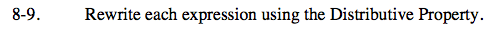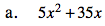What is a common factor between the two terms?

Factor out 5x from both terms.

5x(x + 7)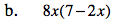Multiply both of the terms in the parentheses by what lies on the outside.

56x − 16x2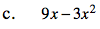Follow the steps in part (a).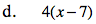Follow the steps in part (b).

4x − 28